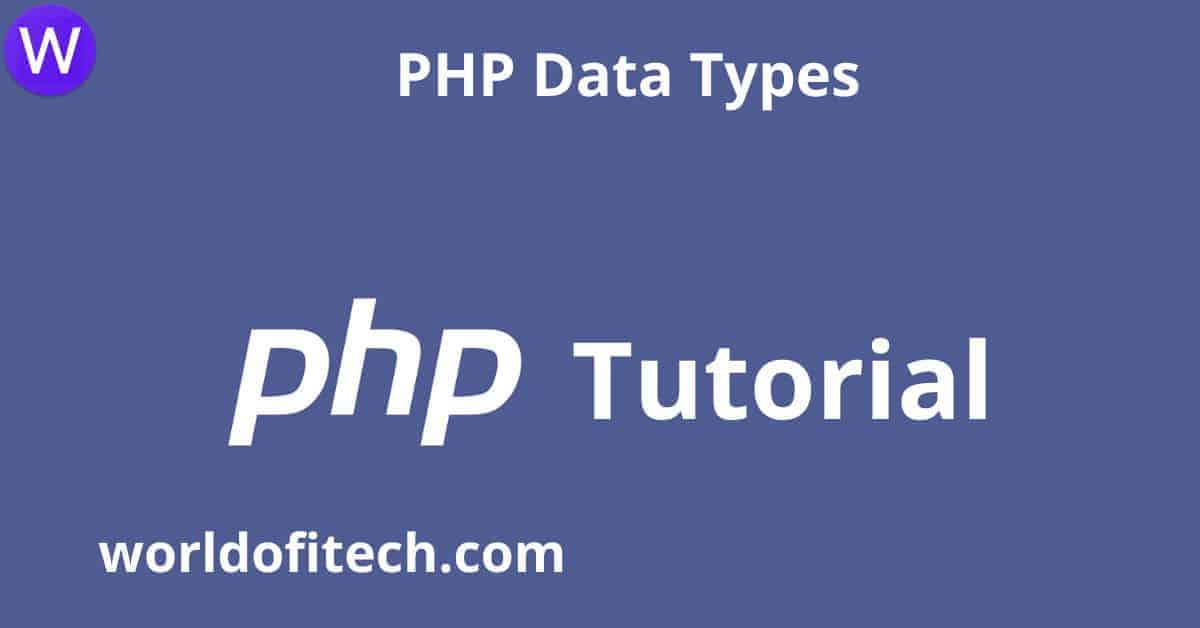# PHP Data TypesIn this tutorial, you will learn about PHP Data Types step by step. So without much to do, let’s get started.

In this tutorial, you will learn-

Contents

## PHP Data Types

Variables can store data of different types, and different data types can do different things.

PHP supports the following data types:

• String
• Integer
• Float (floating point numbers – also called double)
• Boolean
• Array
• Object
• NULL
• Resource

## PHP String

A string is a sequence of characters, like “Hello world!”.

A string can be any text inside quotes. You can use single or double quotes:

Example

```<!DOCTYPE html>
<html>
<body>

<?php
\$x = "Hello world!";
\$y = 'Hello world!';

echo \$x;
echo "<br>";
echo \$y;
?>

</body>
</html>
```

## PHP Integer

An integer data type is a non-decimal number among -2,147,483,648 and 2,147,483,647.

Rules for integers:

• An integer must have at least one digit
• An integer must no longer have a decimal point
• An integer can be either positive or negative
• Integers can be specified in: decimal (base 10), hexadecimal (base 16), octal (base 8), or binary (base 2) notation

In the following instance \$x is an integer. The PHP var_dump() function returns the data type and value:

Example

```<!DOCTYPE html>
<html>
<body>

<?php
\$x = 5985;
var_dump(\$x);
?>

</body>
</html>
```

## PHP Float

A float (floating point number) is a number with a decimal point or a number in exponential form.
In the following example \$x is a float. The PHP var_dump() function returns the data type and value:

Example

```<!DOCTYPE html>
<html>
<body>

<?php
\$x = 10.365;
var_dump(\$x);
?>

</body>
</html>
```

## PHP Boolean

A Boolean represents two possible states: TRUE or FALSE.

\$x = true;
\$y = false;

Booleans are often used in conditional testing. You will learn more about conditional testing in a later chapter of this tutorial.

## PHP Array

An array stores multiple values in one single variable.

In the following example \$cars is an array. The PHP var_dump() function returns the data and value:

Example

```<!DOCTYPE html>
<html>
<body>

<?php
\$cars = array("Volvo","BMW","Toyota");
var_dump(\$cars);
?>

</body>
</html>
```

You will learn a lot more about arrays in later chapters of this tutorial.

## PHP Object

Classes and objects are the two main aspects of object-orientated programming.

A class is a template for objects, and an object is an instance of a class.

When the individual object are created, they inherit all the properties and behaviors from the class, however each object will have different values for the properties.

Let’s assume we have a class named Car. A Car can have properties like model, color, and many others. We can define variables like \$model, \$color, and so on, to maintain the values of these properties.

When the individual object (Volvo, BMW, Toyota, and so forth.) are created, they inherit all of the properties and behaviors from the class, but each object will have different values for the properties.

If you create a __construct() function, PHP will automatically call this function when you create an object from a class.

Example

```<!DOCTYPE html>
<html>
<body>

<?php
class Car {
public \$color;
public \$model;
public function __construct(\$color, \$model) {
\$this->color = \$color;
\$this->model = \$model;
}
public function message() {
return "My car is a " . \$this->color . " " . \$this->model . "!";
}
}

\$myCar = new Car("black", "Volvo");
echo \$myCar -> message();
echo "<br>";
\$myCar = new Car("red", "Toyota");
echo \$myCar -> message();
?>

</body>
</html>
```

## PHP NULL Value

Null is a special data type that could have only one value: NULL.

A variable of data type NULL is a variable that has no value assigned to it.

Tip: If a variable is created with out a value, it is automatically assigned a value of NULL.

Variables can also be emptied by setting the value to NULL:

Example

```<!DOCTYPE html>
<html>
<body>

<?php
\$x = "Hello world!";
\$x = null;
var_dump(\$x);
?>

</body>
</html>
```

## PHP Resource

The special resource type is not an actual data type. It is the storing of a reference to functions and resources external to PHP.

A common example of using the resource data type is a database call.

We will now not talk about the useful resource type here, since it is an advanced topic.

PHP Installation

PHP Syntax

PHP Variables

PHP Variables Scope

This is about PHP Data Types, and we hope you have learned something from this tutorial and share your opinion about this tutorial. What do you think about it, and if you think this tutorial will help some of your friends, do share it with them.### PHP echo and print Statements### PHP Strings